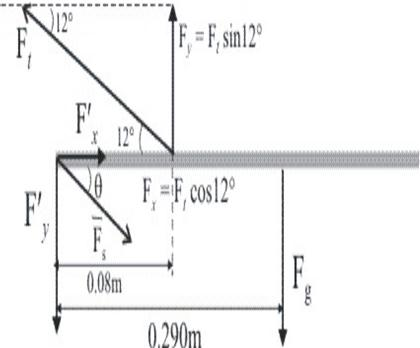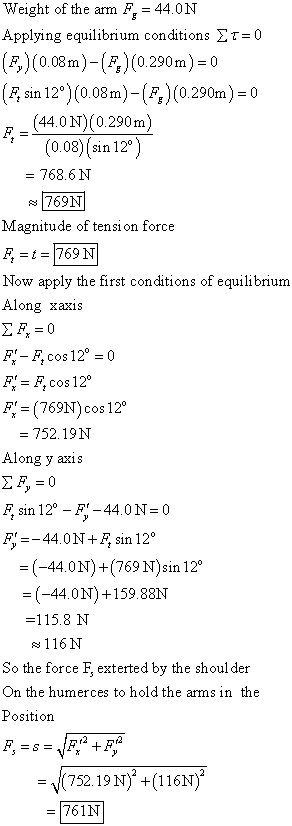# The arm weighs 44.0 N. The force of gravity acting on the arm acts through point A. Determine the magnitudes of the tension force t in the deltoid muscle and the force s of the shoulder on the humerus (upper-arm bone) to hold the arm in the position shown. t=N s=NpreprekomW 2021-04-21 Answered
The arm weighs 44.0 N. The force of gravity acting on the arm acts through point A. Determine the magnitudes of the tension force t in the deltoid muscle and the force s of the shoulder on the humerus (upper-arm bone) to hold the arm in the position shown.
t=N
s=N
You can still ask an expert for help

• Questions are typically answered in as fast as 30 minutes

Solve your problem for the price of one coffee

• Math expert for every subject
• Pay only if we can solve itunett
Here is a photo:###### Not exactly what you’re looking for?Jeffrey Jordon

Weight of the arm

Applying equlibrium conditions $\sum \tau =0$

Magnitude of tension force

Now apply the first conditions of equlibtium

Along xaxis

$\sum {F}_{x}=0$

${F}_{x}^{\prime }-{F}_{t}\mathrm{cos}{12}^{\circ }=0$

${F}_{x}^{\prime }={F}_{t}\mathrm{cos}{12}^{\circ }$

Along y axis

$\sum {F}_{y}=0$

So the force F, extered by the shoulder. On the humerces to hold the arms in the position

${F}_{s}=s=\sqrt{{F}_{x}^{\prime 2}+{F}_{y}^{\prime 2}}$